Name:    Chapter 19 Online Practice

Multiple Choice
Identify the letter of the choice that best completes the statement or answers the question.

1.

Reactants A and B are placed in an empty flask. If the reaction A(g) + B(g) f 2 C(g) has Kp > 1, which of the following statements are correct?

 1. The forward reaction is spontaneous. 2. The forward rate of reaction is fast. 3. The reaction is reactant-favored.
 a. 1 only b. 2 only c. 3 only d. 1 and 2 e. 1, 2, and 3

2.

The following processes occur spontaneously at 25 °C. Which of these processes are endothermic?

 1. the combustion of methane to produce water and carbon dioxide 2. the expansion of an ideal gas into a vacuum 3. the melting of ice
 a. 1 only b. 2 only c. 3 only d. 1 and 2 e. 2 and 3

3.

A statement of the second law of thermodynamics is that
 a. spontaneous reactions are always exothermic. b. energy is conserved in a chemical reaction. c. the Gibbs free energy is a function of both enthalpy and entropy. d. DS = - DH for any chemical reaction. e. in a spontaneous process, the entropy of the universe increases.

4.

As defined by Ludwig Boltzmann, the third law of thermodynamics states that
 a. in a spontaneous process, the entropy of the universe increases. b. there is no disorder in a perfect crystal at 0 K. c. the total entropy of the universe is always increasing. d. the total energy of the universe is constant. e. mass and energy are conserved in all chemical reactions.

5.

Which of the following linear chain alcohols is likely to have the highest standard entropy in the liquid state?
 a. CH3OH b. CH3CH2OH c. CH3CH2CH2OH d. CH3CH2CH2CH2OH e. CH3CH2CH2CH2CH2OH

6.

Which of the following processes are reversible?

 1. the expansion of an ideal gas into a vacuum 2. the melting of ice at 0 °C 3. the combustion of carbon to produce carbon dioxide
 a. 1 only b. 2 only c. 3 only d. 1 and 2 e. 2 and 3

7.

If a chemical reaction has a positive change in entropy then
 a. the change in enthalpy is negative. b. the reaction is exothermic. c. heat goes from the system into the surroundings. d. the disorder of the system increases. e. the reaction is spontaneous.

8.

Which of the following processes involves a decrease in entropy?
 a. the decomposition of NH3(g) into H2(g) and N2(g) gas b. the dissolution of NaCl in water c. the condensation of steam to liquid water d. the evaporation of ethanol e. the sublimation of dry ice (i.e., CO2(s))

9.

All of the following statements concerning entropy are true EXCEPT
 a. entropy values for substances are greater than or equal to zero. b. entropy is a state function. c. a positive change in entropy denotes a change toward greater disorder. d. entropy is zero for elements under standard conditions. e. the entropy of a substance in the gas phase is greater than the solid phase.

10.

Which reaction is likely to have a negative change in entropy?
 a. 2 NH3(g) ® N2(g) + 3 H2(g) b. CaCO3(s) ® CaO(s) + CO2(g) c. NaCl(s) ® Na+(aq) + Cl-(aq) d. N2O4(g) ® 2 NO2(g) e. 2 CO(g) ® 2 C(s) + O2(g)

11.

Calculate the standard entropy change for the combustion of methanol at 25 °C.

2 CH3OH(l) + 3 O2(g) ® 2 CO2(g) + 4 H2O(g)

 Species S° (J/K×mol) CH 3 OH (l) 127.2 O 2 (g) 205.1 CO 2 (g) 213.7 H 2 O(g) 188.8
 a. -2052.3 J/K b. -312.9 J/K c. +70.2 J/K d. +312.9 J/K e. +2052.3 J/K

12.

The standard entropy of formation of PCl3(g) is -33.2 J/K·mol.

1/4 P4(s) + 3/2 Cl2(g) ® PCl3(g)

Calculate the standard molar entropy of PCl3(g) given S°[P4(s)] = 41.1 J/K·mol and S°[Cl2(g)] = 223.1 J/K·mol.
 a. -378.1 J/K·mol b. -297.4 J/K·mol c. +212.2 J/K·mol d. +231.0 J/K·mol e. +311.7 J/K·mol

13.

Predict the signs of DH and DS for the condensation of steam at 85 °C.
 a. DH < 0 and DS < 0 b. DH < 0 and DS > 0 c. DH > 0 and DS < 0 d. DH > 0 and DS > 0 e. DH = 0 and DS < 0

14.

Hydrogen gas is a non-polluting fuel. Predict the signs of DH, DS, and DG for the combustion of hydrogen gas at 150 °C.

2 H2(g) + O2(g) ® 2 H2O(g)
 a. DH < 0, DS > 0, DG < 0 b. DH < 0, DS < 0, DG < 0 c. DH < 0, DS > 0, DG < 0 d. DH > 0, DS < 0, DG < 0 e. DH > 0, DS < 0, DG > 0

15.

If DG° < 0 for a reaction at all temperatures, then DH° is ____ and DS° is ____.
 a. negative, positive b. positive, negative c. negative, negative d. positive, positive e. positive, either positive or negative

16.

The dissolution of ammonium nitrate occurs spontaneously in water at 25 °C. As NH4NO3 dissolves, the temperature of the water decreases. What are the signs of DH, DS, and DG for this process?
 a. DH > 0, DS < 0, DG > 0 b. DH > 0, DS > 0, DG > 0 c. DH > 0, DS > 0, DG < 0 d. DH < 0, DS < 0, DG < 0 e. DH < 0, DS > 0, DG > 0

17.

At what temperatures will a reaction be spontaneous if DH = +158 kJ and DS = +411 J/K?
 a. All temperatures below 384 K b. Temperatures between 158 K and 411 K c. All temperatures above 384 K d. The reaction will be spontaneous at any temperature. e. The reaction will never be spontaneous.

18.

If a process is exothermic and spontaneous, which of the following must be true?
 a. DG > 0 and DH < 0 b. DG < 0 and DH < 0 c. DG < 0 and DS > 0 d. DH < 0 and DS > 0 e. DH > 0 and DS < 0

19.

Calculate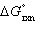for the reaction below at 25.0 °C

2 H2O(g) + S(s) ® 2 H2S(g) + O2(g)

given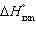= +442.4 kJ and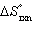= +206.9 J/K.
 a. +380.7 kJ b. +504.1 kJ c. +649.3 kJ d. +1277.7 kJ e. +6.125 ´ 104 kJ

20.

All of the following substances have a standard free energy of zero EXCEPT
 a. Br2(l). b. I2(g). c. S8(s). d. Cl2(g). e. Hg(l).

21.

Thermodynamics can be used to determine all of the following EXCEPT
 a. the rate of reaction. b. the extent to which a reaction occurs. c. the direction in which a reaction is spontaneous. d. the temperature at which a reaction is spontaneous. e. the entropy change of a reaction.

22.

For a chemical reaction, if DG° = 0, then
 a. K > 1 b. K = 0 c. K < 1 d. K < 0 e. K = 1

23.

All of the following relationships are true EXCEPT
 a.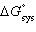=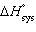- T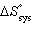b.= -RT ln (K) c.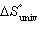=+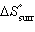d. DH =+ RT ln (K) e.= -T24.

For a chemical system, DG° and DG are equal when
 a. the system is in equilibrium. b. the reactants and products are in standard state conditions. c. the equilibrium constant, K, equals 0. d. the reaction quotient, Q, is less than 1. e. the reactants and products are in the gas phase.

25.

The standard free energy change for a chemical reaction is -18.3 kJ/mole. What is the equilibrium constant for the reaction at 87 °C? (R = 8.314 J/K·mol)
 a. 2.2 ´ 10-3 b. 1.0 c. 6.1 d. 4.5 ´ 102 e. 1.3 ´ 106

26.

The equilibrium constant for a reaction at 25 °C is 4.7 ´ 10-8. What is DG°? (R = 8.314 J/K·mol)
 a. +1.52 kJ b. +3.51 kJ c. +6.81 kJ d. +18.2 kJ e. +41.8 kJ